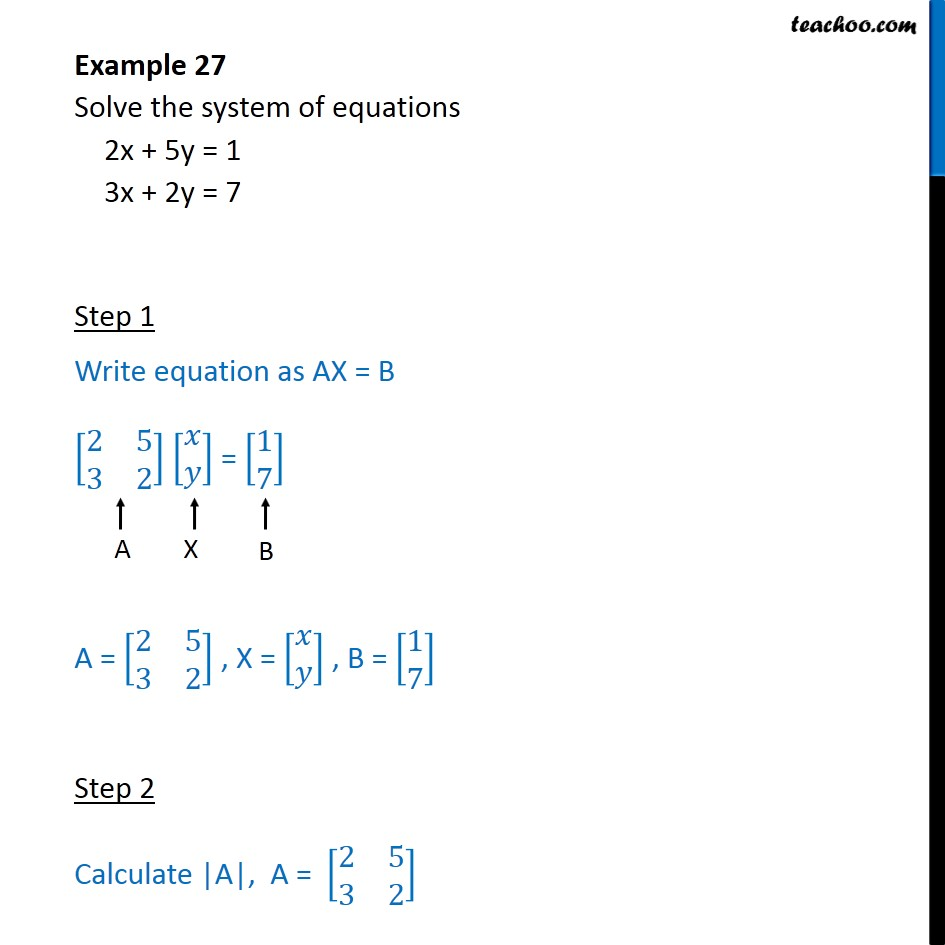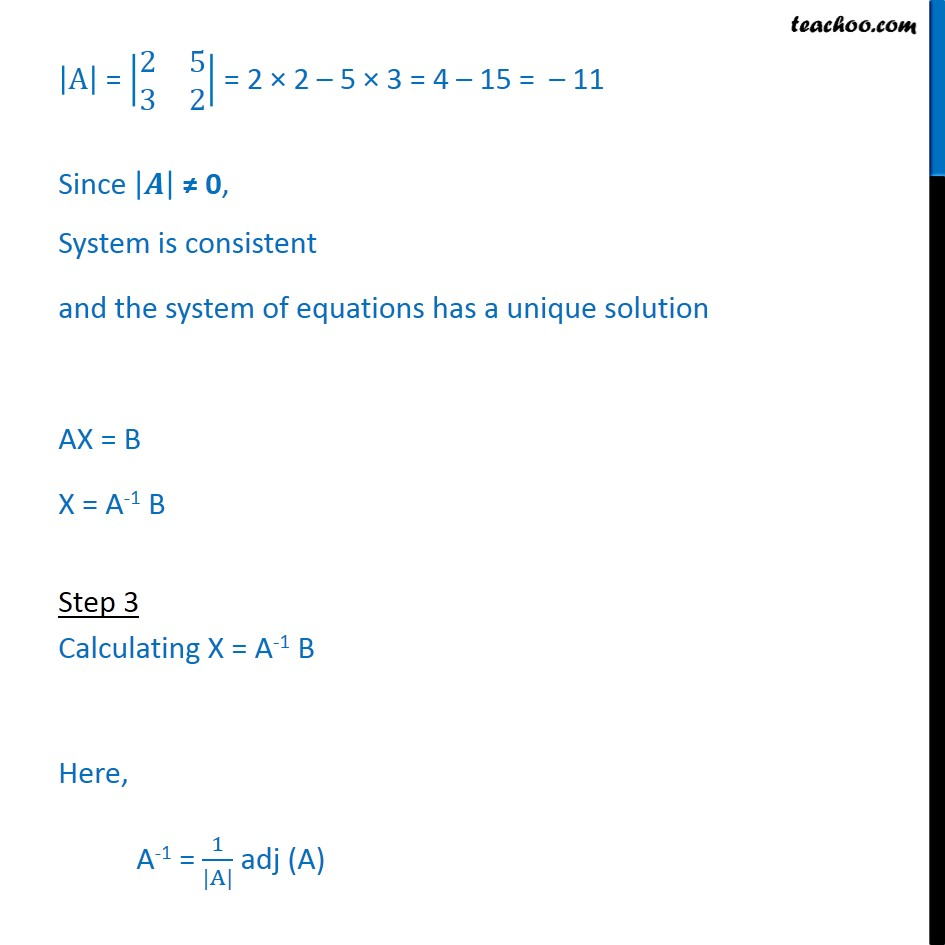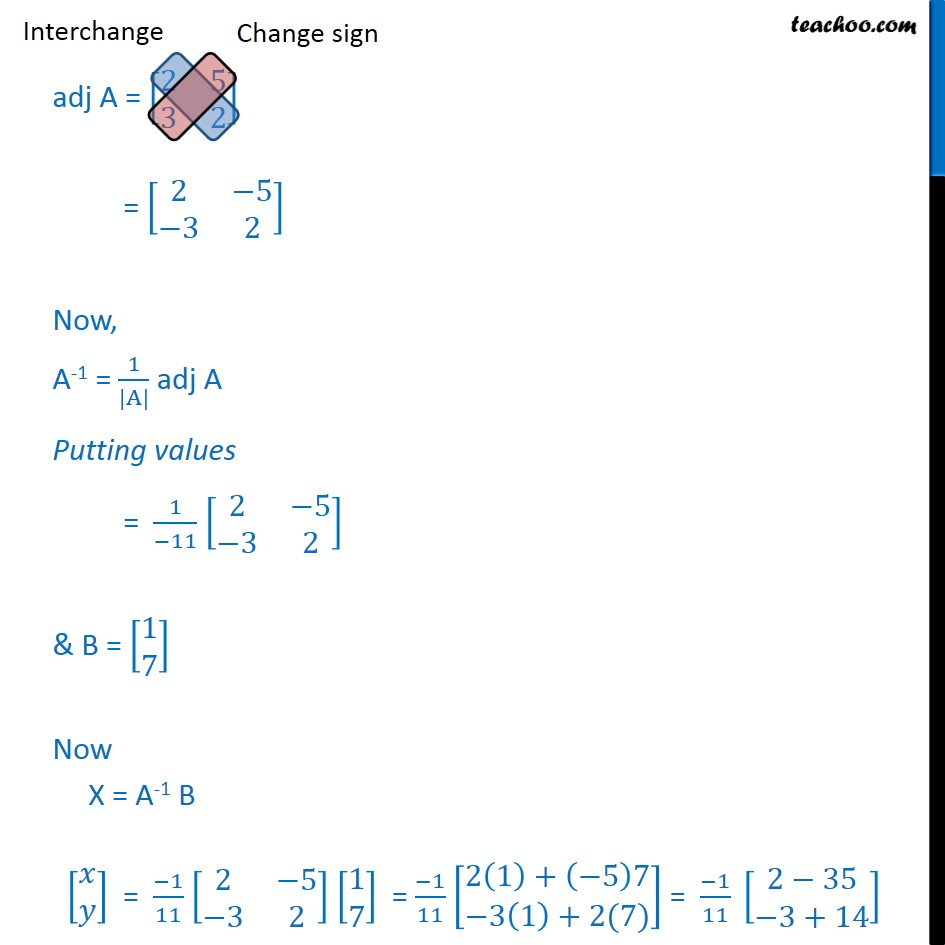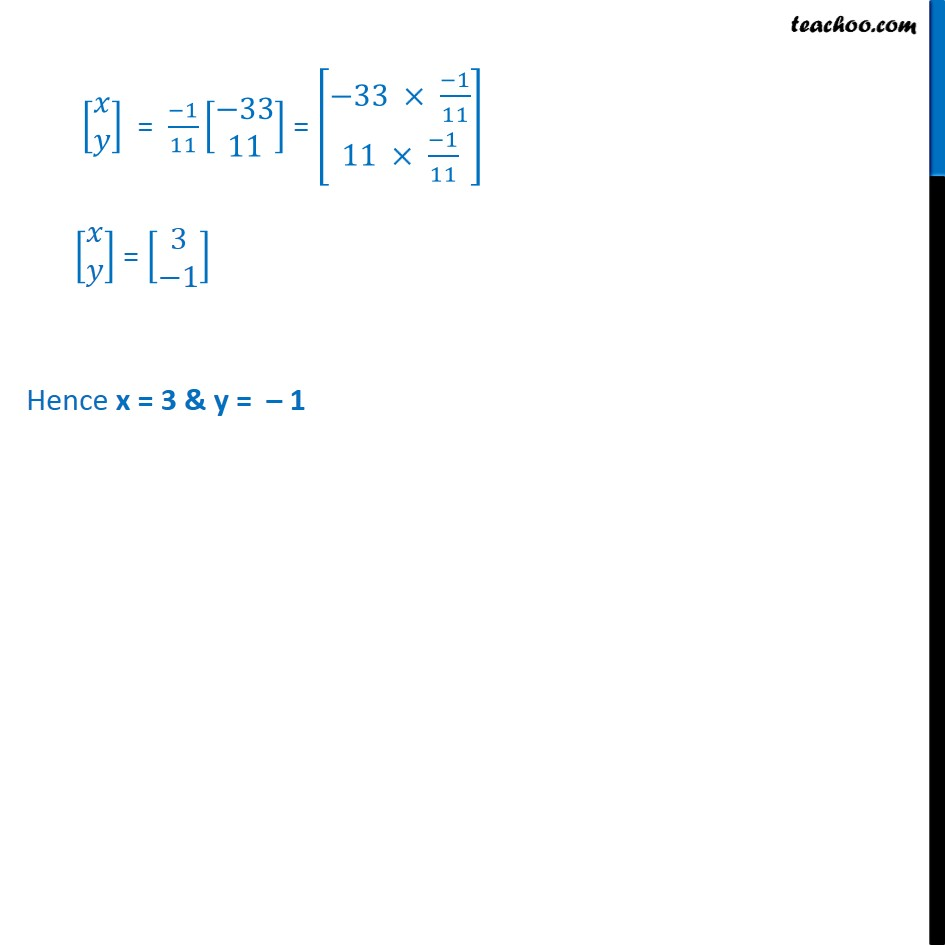Find solution of equations- Equations given

Chapter 4 Class 12 Determinants
Concept wiseLearn in your speed, with individual attention - Teachoo Maths 1-on-1 Class

### Transcript

A﷯ = 2﷮5﷮3﷮2﷯﷯ = 2 × 2 – 5 × 3 = 4 – 15 = – 11 Since 𝑨﷯ ≠ 0, System is consistent and the system of equations has a unique solution AX = B X = A-1 B Step 3 Calculating X = A-1 B Here, A-1 = 1﷮|A|﷯ adj (A) adj A = 2﷮5﷮3﷮2﷯﷯ = 2﷮−5﷮−3﷮2﷯﷯ Now, A-1 = 1﷮|A|﷯ adj A Putting values = 1﷮−11﷯ 2﷮−5﷮−3﷮2﷯﷯ & B = 1﷮7﷯﷯ Now X = A-1 B 𝑥﷮𝑦﷯﷯ = −1﷮11﷯ 2﷮−5﷮−3﷮2﷯﷯ 1﷮7﷯﷯ = −1﷮11﷯ 2 1﷯+ −5﷯7﷮−3 1﷯+2(7)﷯﷯ = −1﷮11﷯ 2−35﷮−3+14﷯﷯ 𝑥﷮𝑦﷯﷯ = −1﷮11﷯ −33﷮11﷯﷯ = −33 × −1﷮11﷯﷮11 × −1﷮11﷯﷯﷯ 𝑥﷮𝑦﷯﷯ = 3﷮−1﷯﷯ Hence x = 3 & y = – 1